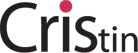Ph.D. started in: 2015
Expected year of graduation: 2019
COINS consortium member: University of Bergen
Supervised by: Håvard Raddum
Links:Research area: Cryptography
Project title: Application of BDD to Cryptography
Project description: In Algebraic Cryptanalysis, one creates equation system of the cipher being analysed. Solving this equation system reveal some secret information about the system. There are several ways to represent the equation system such as ANF (Algebraic Normal Form), CNF, MRHS (Multiple Right Hand Side) CRHS (Compressed Right Hand Side). The system of equation can be both linear , non-linear or combination of both linear and non-linear terms. Binary Decision Diagrams helps in solving non-linear equations there by decreasing the time complexity

Events attended with COINS funding:
1. COINS Finse winter school , Finse, Norway, 2016
2. COINS Ph.D. student seminar, Bergen, Norway, 2016
This entry was posted in Students and tagged . Bookmark the permalink.